# How is the electrical charge related to time?

## Amperage, voltage and resistance¶

In order for an electric current to flow through a body, two conditions must be met:

• Freely movable charge carriers (for example electrons) must be present in it.
• An electrical voltage (charge difference) must be applied to it.

Model:

Similar to how water can be set in motion by a pump, electrons can be set in motion by power sources ("electron pumps").

While water is pressed into a water pipe with a water pump, a power source (battery or generator) generates an increased concentration of electrons at one of its poles and thus an electrical voltage.

### The amperage¶

An electric current occurs when electric charges move. The electrical current is a measure of the amount of electrical charge that flows through a body in a given period of time.

Model:

When a tap is turned on, water begins to flow. The further the tap is turned on, the more water flows out of it in a certain time. We could call this parameter “water flow strength”.

Correspondingly, the orderly movement of electrical charges leads to a flow of electrical current: the more electrons (or ions) pass through a conductor in a certain time, the higher the electrical current. Just as the maximum amount of water that can flow in a water pipe depends on the diameter of the water pipe, a stronger current can flow in a thick conductor than in a thin one.

Definition:

The electric current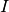is equal to the amount of electrical charge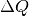that in a span of time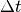is transported:

Unit:

The electric current is in amperes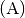specified. A current of 1 ampere means that 1 coulomb of charge (about 6 trillion electrons!) Is transported per second at every point in the conductor.

Physical and technical current direction

The movement of charge carriers could be observed for the first time in electrically conductive liquids ("electrolytes") in the form of positively charged ions. Based on this historical discovery, the current direction was defined as the direction along which positively charged charge carriers move in a conductor: They always start from the plus pole of a power source and move in the direction of the minus pole.

This convention was retained, although it was later found that in conductive solids, the movement of negatively charged electrons is of decisive importance for the flow of current. Since these start from the minus pole and move in the direction of the plus pole, a distinction must always be made between the originally determined ("technical") and the actual ("physical") direction of movement of the charged particles:

• The "technical" direction of current always runs from plus to minus.
• The “physical” direction of the current indicates the actual direction of movement of the charged particles. For positive charge carriers it is identical to the technical current direction, for negative charge carriers it points in the opposite direction.

In today's electronics, the technical direction of current is always used as the standard for circuit diagrams and labels. Even if the charge is transported by electrons, it is said that the current flows “from plus to minus”. This may be physically incorrect; What is more important, however, is that components that are dependent on polarity (e.g. diodes and transistors) are always manufactured according to the same scheme. 

Current density

In addition to the amperageis sometimes also used in electrical engineering with the so-called current density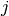expected. This results from the amperageby the cross-sectional area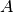of the conductor divided:

The unit for the current density is usually chosen on the basis of the common conductor cross-sections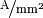. The current density can be used to explain, for example, why the wire in a lightbulb shines, but not the supply lines: Although the current strength is the same, the current density and thus the heat development is higher in the much thinner wire inside the lightbulb.

### The electrical voltage¶

Electric current does not flow by itself, but requires an electric voltage as the cause. Electric voltage, in turn, is the result of a charge separation, for example an increase in the concentration of electrons at one point compared to another.

Model:

In a water tank, the pressure exerted on the tap depends exclusively on the filling level in the tank: the higher the tank is filled, the higher the water pressure at the lower end.

Correspondingly, a targeted accumulation of electrical charge is necessary for an electrical current to flow. The more the electrons are compressed at one point, i.e. the more work is invested in separating the charge, the steeper the concentration gradient of electrical charge and thus the electrical voltage.

Definition:

The electrical voltage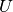is equal to the amount of work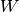which, when the charge is separated, apply to an amount of charge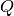is expended:

Unit:

The electrical potential

The tension is not an absolute quantity, so strictly speaking it is not possible to state how great the tension is at a certain point. Rather, tension always relates to two points: a tension value can only be used to indicate how great the tension is between two points is. According to the technical convention that current always flows “from plus to minus”, the voltage difference between two points can be viewed as a measure of how strongly and in which direction the current wants to flow from one point to the other.

In order to be able to give an absolute value for the amount of a charge accumulation, one has the term "electrical potential"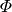introduced. The electrical potential is comparable to the height of a point above sea level. Such a value can actually be given for each individual point. If one then compares two different points with regard to their electrical potentials, the difference can be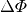these potentials as electrical voltagebe understood between the two points:

In everyday parlance, despite this difference, the terms voltage and electrical potential are often used as equivalent. The reason for this is that the negative connection of the power source is often used as the reference point (“earth”, “ground”, “zero potential”) for the electrical potential and this is arbitrarily given the value zero. Compared to this reference point, the electrical potential of a point is identical to the voltage value. If, on the other hand, two points (for example in an electronic circuit) each have electrical potentials different from zero, only the difference between the respective electrical potentials (colloquially: "voltage difference") is important as the cause of a current flow.

### The DC voltage¶

An electrical direct voltage is created when an excess of electrical charge is generated at one point by a power source. If the circuit is closed, the freely moving electrons in the conductor flow from the point with the higher to the point with the lower charge concentration in order to bring about a charge equalization.

A DC voltage that is constant over time causes a current that is constant over time ("DC current") in a conductor.  However, it is also referred to as a direct voltage if it assumes values ​​of different sizes over time, but always maintains the same polarity. In this case, the result is a so-called “pulsating direct current”.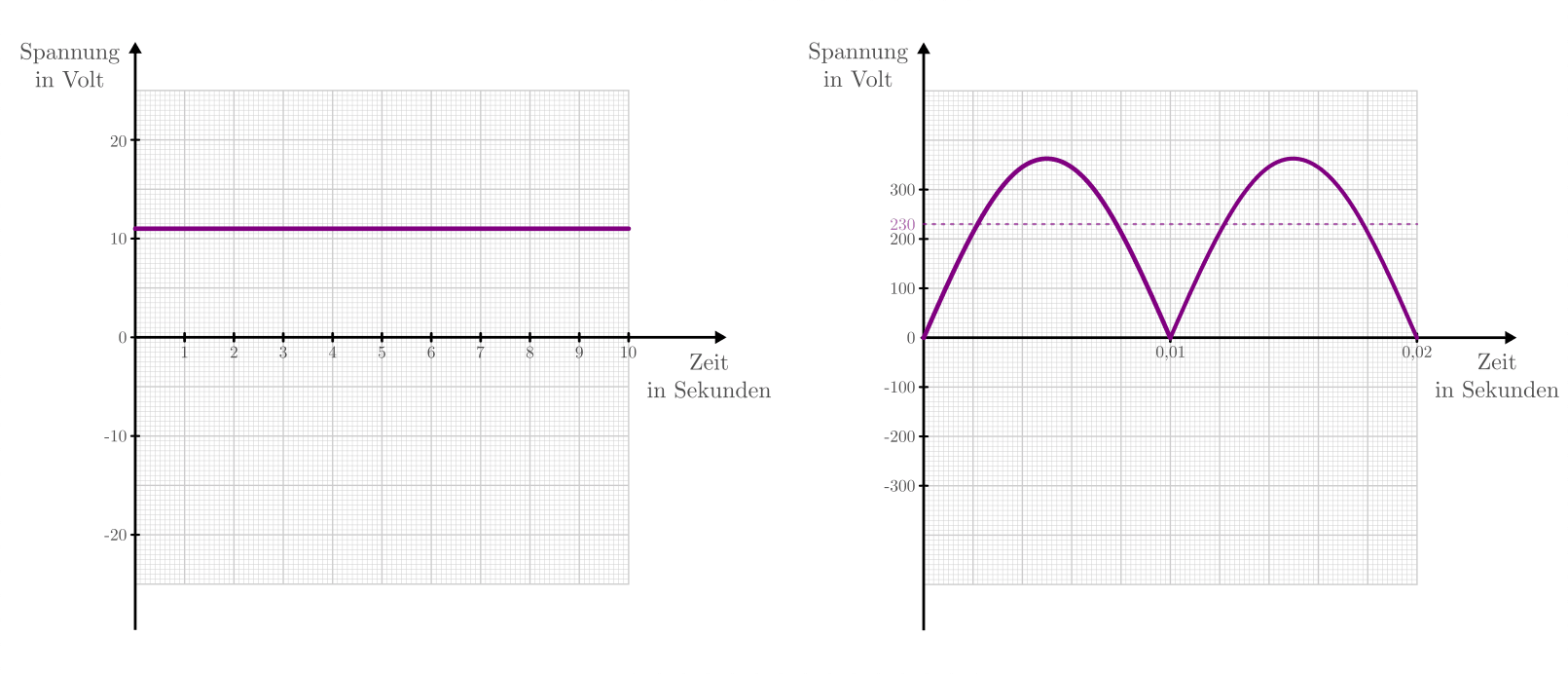Diagrams of a "real"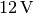-Dc voltage and a "pulsating"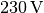DC voltage.

### The alternating voltage¶

Some electrical generators, for example bicycle dynamos, produce a current that flows once "from right to left" and then again "from left to right".  The reason for this is a voltage that changes direction (or its sign) regularly and is therefore referred to as alternating voltage.

The best-known alternating voltage is the sinusoidal voltage of the power grid, which is periodically between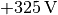and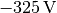changes back and forth. Due to the fluctuations, however, only as much electrical power can be converted as with a DC voltage of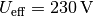.  This so-called "effective voltage" of a sinusoidal alternating voltage can generally be calculated using the following formula: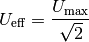The frequency of the alternating voltage in the power grid is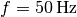; The alternating voltage runs through in one second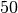Periods. This results in an oscillation period of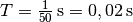.

The advantage of an alternating voltage over a (temporally constant) direct voltage is that the value of the alternating voltage can easily be set to any other voltage value using a suitable transformer.

### The electrical resistance¶

The charge carriers can move freely in an ideal electrical conductor. In a real conductor, on the other hand, there are always interactions between the moving electrons and the remaining atomic cores. Similar to mechanical friction, electrical energy is converted into thermal energy (see also the section on heat generation in electrical components).

With poor conductors (e.g. iron), the interaction between electrons and atoms is stronger than with good conductors (e.g. copper). On the one hand, bad conductors heat up faster than good conductors, and on the other hand, a higher electrical voltage must be applied to them in order to achieve the same current flow.

Definition:

Under the electrical resistance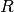of a circuit is understood to be the relationship between the voltagebetween the ends of a conductor and the strength of the currentin the head:

The electrical resistance of individual components can also be specified in the same way.

Unit: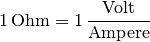### Ohm's law¶

The relationship between electrical voltage, current strength and resistance was discovered in 1826 by Georg Simon Ohm. In his honor, the resistance equation (5) is still called "Ohm's law" and is given in the following form:

Ohm's law (6) is limited in its validity to homogeneous materials. In numerous cases, however, for example with series and parallel connections, it can help to determine voltages or currents if the respective electrical resistances are known.

In addition to the two previously mentioned forms (5) and (6), the relationship between current strength and voltage can also be specified as follows: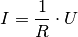With a higher voltage, the current that flows through a circuit or an electronic component is also higher. As can be seen in Ohm's law, the corresponding characteristic curve corresponds to a straight line that is steeper, the lower the resistance valueis.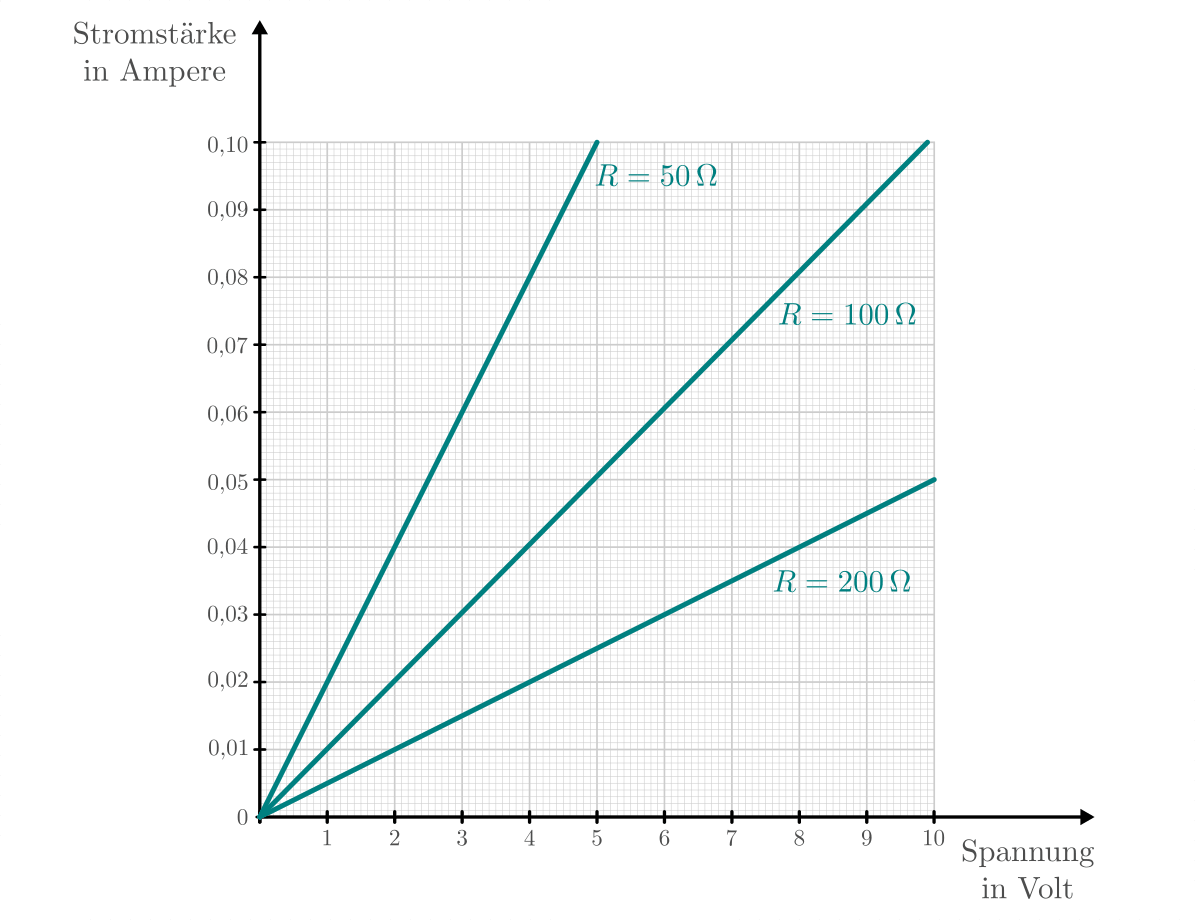The current intensity as a function of the voltage with fixed resistance values ​​(Ohm's law).

In electronics, components that at least approximately fulfill these characteristics are also referred to as resistors.

Non-ohmic resistances

In semiconductor components (e.g. diodes, transistors, etc.) the relationship between current strength and voltage is not linear; rather, a current flow is only possible in these components above a certain voltage, which increases disproportionately with a further increase in voltage (figure characteristic curve of a diode in forward direction).

Ohm's law can be used (approximately) in such cases if the resistance values ​​are known as a function of the current strength or voltage, i.e. are available as a diagram or in tabular form for certain values.

### The specific resistance¶

Different substances conduct electricity differently, so they have different specific resistance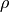. The lower the specific resistance of a material, the better it conducts electrical current. 

Definition:

In the case of a body made of homogeneous material, for example a metal wire, the resistance at a certain temperature depends on the material, on its lengthand its cross-sectional area ("thickness")from. The following applies:

Unit:

From the definition (7) results for the specific resistancethe unit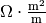.

Since most components and lines have a much smaller cross-sectional area than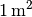the following unit is also common: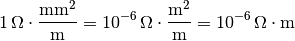Examples:

Since the specific resistances of different substances differ considerably from one another, the materials can be divided into electrical conductors, semiconductors and insulators.

Temperature dependence of the specific resistance

In the case of metallic conductors, the specific resistance dependslinearly from the temperature over a wide temperature range: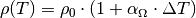This is called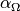as the temperature coefficient of ohmic resistances. For metals is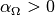, the specific resistance thus increases with increasing temperature. Semiconductors, on the other hand, become more conductive with increasing temperatures, while at low temperatures they increasingly become insulators.

Annotation: